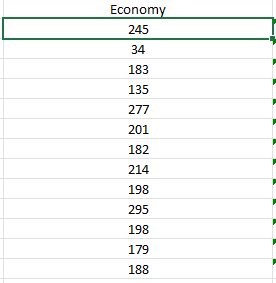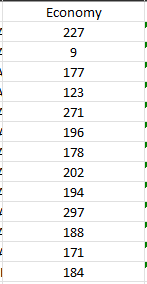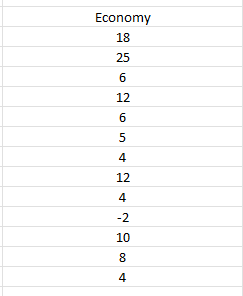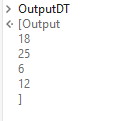# Comparing 2 Datatable

Hello Everyone,

I have 2 different excel files and I want to compare the columns in this excel. For example, 1. excel 1. row value is 200 and 2. excel 1. row value is 20. I want to subtract 20 from 200 and create a 3. excel file and write to it.

First Excel:Second Excel:Output excel that i want:You can build a datatable with 3 columns. Load the data from 2 excels to first 2 columns & use the following query in the Invoke Code activity to fill the 3rd column:

``````dt.AsEnumerable.ToList.ForEach(Sub(row)
row(2) = (CInt(row(0).ToString) - CInt(row(1).ToString))
End Sub)
``````

Hope this helps,
Best Regards.

2 Likes

@Atakan_Genc

1. Read data from Excels into two tables dt1 and dt2
2. use build datatable and have one column with integer type and assign to dt3
3. Use for each row in datatable on dt1 and there is a index property in ‘for’ properties assign a variable to it
4. Use Add data row activity with {Cint(currentrow(0).ToString)-Cint(dt.Rows(indexvar)(0).ToString)} and give dt3 in datatable
5. Write dt3 to and excel using write range

Hope this helps

cheers

1 Like

Hey @Atakan_Genc ,